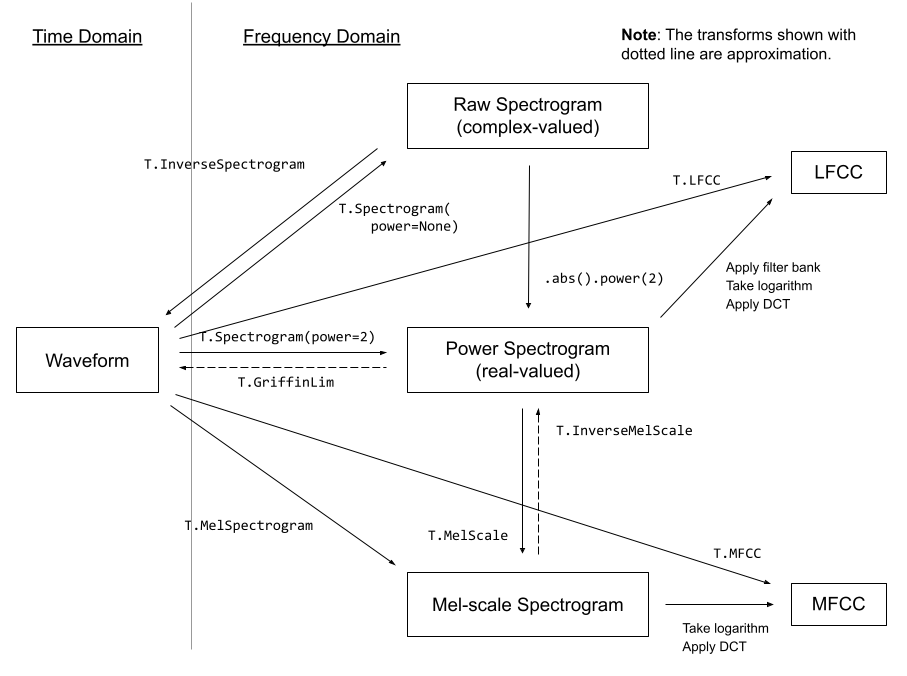Shortcuts

# Audio Feature Extractions¶

Author: Moto Hira

torchaudio implements feature extractions commonly used in the audio domain. They are available in torchaudio.functional and torchaudio.transforms.

functional implements features as standalone functions. They are stateless.

transforms implements features as objects, using implementations from functional and torch.nn.Module. They can be serialized using TorchScript.

import torch
import torchaudio
import torchaudio.functional as F
import torchaudio.transforms as T

print(torch.__version__)
print(torchaudio.__version__)

2.1.0.dev20230604
2.1.0.dev20230604


## Preparation¶

Note

When running this tutorial in Google Colab, install the required packages

!pip install librosa

from IPython.display import Audio
import librosa
import matplotlib.pyplot as plt

torch.random.manual_seed(0)

def plot_waveform(waveform, sr, title="Waveform"):
waveform = waveform.numpy()

num_channels, num_frames = waveform.shape
time_axis = torch.arange(0, num_frames) / sr

figure, axes = plt.subplots(num_channels, 1)
axes.plot(time_axis, waveform, linewidth=1)
axes.grid(True)
figure.suptitle(title)
plt.show(block=False)

def plot_spectrogram(specgram, title=None, ylabel="freq_bin"):
fig, axs = plt.subplots(1, 1)
axs.set_title(title or "Spectrogram (db)")
axs.set_ylabel(ylabel)
axs.set_xlabel("frame")
im = axs.imshow(librosa.power_to_db(specgram), origin="lower", aspect="auto")
fig.colorbar(im, ax=axs)
plt.show(block=False)

def plot_fbank(fbank, title=None):
fig, axs = plt.subplots(1, 1)
axs.set_title(title or "Filter bank")
axs.imshow(fbank, aspect="auto")
axs.set_ylabel("frequency bin")
axs.set_xlabel("mel bin")
plt.show(block=False)


## Overview of audio features¶

The following diagram shows the relationship between common audio features and torchaudio APIs to generate them.For the complete list of available features, please refer to the documentation.

## Spectrogram¶

To get the frequency make-up of an audio signal as it varies with time, you can use torchaudio.transforms.Spectrogram().

SPEECH_WAVEFORM, SAMPLE_RATE = torchaudio.load(SAMPLE_SPEECH)

plot_waveform(SPEECH_WAVEFORM, SAMPLE_RATE, title="Original waveform")
Audio(SPEECH_WAVEFORM.numpy(), rate=SAMPLE_RATE)n_fft = 1024
win_length = None
hop_length = 512

# Define transform
spectrogram = T.Spectrogram(
n_fft=n_fft,
win_length=win_length,
hop_length=hop_length,
center=True,
power=2.0,
)

# Perform transform
spec = spectrogram(SPEECH_WAVEFORM)

plot_spectrogram(spec, title="torchaudio")## GriffinLim¶

To recover a waveform from a spectrogram, you can use GriffinLim.

torch.random.manual_seed(0)

n_fft = 1024
win_length = None
hop_length = 512

spec = T.Spectrogram(
n_fft=n_fft,
win_length=win_length,
hop_length=hop_length,
)(SPEECH_WAVEFORM)

griffin_lim = T.GriffinLim(
n_fft=n_fft,
win_length=win_length,
hop_length=hop_length,
)

reconstructed_waveform = griffin_lim(spec)

plot_waveform(reconstructed_waveform, SAMPLE_RATE, title="Reconstructed")
Audio(reconstructed_waveform, rate=SAMPLE_RATE)## Mel Filter Bank¶

torchaudio.functional.melscale_fbanks() generates the filter bank for converting frequency bins to mel-scale bins.

Since this function does not require input audio/features, there is no equivalent transform in torchaudio.transforms().

n_fft = 256
n_mels = 64
sample_rate = 6000

mel_filters = F.melscale_fbanks(
int(n_fft // 2 + 1),
n_mels=n_mels,
f_min=0.0,
f_max=sample_rate / 2.0,
sample_rate=sample_rate,
norm="slaney",
)

plot_fbank(mel_filters, "Mel Filter Bank - torchaudio")### Comparison against librosa¶

For reference, here is the equivalent way to get the mel filter bank with librosa.

mel_filters_librosa = librosa.filters.mel(
sr=sample_rate,
n_fft=n_fft,
n_mels=n_mels,
fmin=0.0,
fmax=sample_rate / 2.0,
norm="slaney",
htk=True,
).T

plot_fbank(mel_filters_librosa, "Mel Filter Bank - librosa")

mse = torch.square(mel_filters - mel_filters_librosa).mean().item()
print("Mean Square Difference: ", mse)Mean Square Difference:  3.934872696751886e-17


## MelSpectrogram¶

Generating a mel-scale spectrogram involves generating a spectrogram and performing mel-scale conversion. In torchaudio, torchaudio.transforms.MelSpectrogram() provides this functionality.

n_fft = 1024
win_length = None
hop_length = 512
n_mels = 128

mel_spectrogram = T.MelSpectrogram(
sample_rate=sample_rate,
n_fft=n_fft,
win_length=win_length,
hop_length=hop_length,
center=True,
power=2.0,
norm="slaney",
onesided=True,
n_mels=n_mels,
mel_scale="htk",
)

melspec = mel_spectrogram(SPEECH_WAVEFORM)

/work/torchaudio/transforms/_transforms.py:580: UserWarning: Argument 'onesided' has been deprecated and has no influence on the behavior of this module.
warnings.warn(

plot_spectrogram(melspec, title="MelSpectrogram - torchaudio", ylabel="mel freq")### Comparison against librosa¶

For reference, here is the equivalent means of generating mel-scale spectrograms with librosa.

melspec_librosa = librosa.feature.melspectrogram(
y=SPEECH_WAVEFORM.numpy(),
sr=sample_rate,
n_fft=n_fft,
hop_length=hop_length,
win_length=win_length,
center=True,
power=2.0,
n_mels=n_mels,
norm="slaney",
htk=True,
)

plot_spectrogram(melspec_librosa, title="MelSpectrogram - librosa", ylabel="mel freq")

mse = torch.square(melspec - melspec_librosa).mean().item()
print("Mean Square Difference: ", mse)Mean Square Difference:  1.291346363174739e-09


## MFCC¶

n_fft = 2048
win_length = None
hop_length = 512
n_mels = 256
n_mfcc = 256

mfcc_transform = T.MFCC(
sample_rate=sample_rate,
n_mfcc=n_mfcc,
melkwargs={
"n_fft": n_fft,
"n_mels": n_mels,
"hop_length": hop_length,
"mel_scale": "htk",
},
)

mfcc = mfcc_transform(SPEECH_WAVEFORM)

plot_spectrogram(mfcc)### Comparison against librosa¶

melspec = librosa.feature.melspectrogram(
y=SPEECH_WAVEFORM.numpy(),
sr=sample_rate,
n_fft=n_fft,
win_length=win_length,
hop_length=hop_length,
n_mels=n_mels,
htk=True,
norm=None,
)

mfcc_librosa = librosa.feature.mfcc(
S=librosa.core.spectrum.power_to_db(melspec),
n_mfcc=n_mfcc,
dct_type=2,
norm="ortho",
)

plot_spectrogram(mfcc_librosa)

mse = torch.square(mfcc - mfcc_librosa).mean().item()
print("Mean Square Difference: ", mse)Mean Square Difference:  0.810401201248169


## LFCC¶

n_fft = 2048
win_length = None
hop_length = 512
n_lfcc = 256

lfcc_transform = T.LFCC(
sample_rate=sample_rate,
n_lfcc=n_lfcc,
speckwargs={
"n_fft": n_fft,
"win_length": win_length,
"hop_length": hop_length,
},
)

lfcc = lfcc_transform(SPEECH_WAVEFORM)
plot_spectrogram(lfcc)## Pitch¶

pitch = F.detect_pitch_frequency(SPEECH_WAVEFORM, SAMPLE_RATE)

def plot_pitch(waveform, sr, pitch):
figure, axis = plt.subplots(1, 1)
axis.set_title("Pitch Feature")
axis.grid(True)

end_time = waveform.shape / sr
time_axis = torch.linspace(0, end_time, waveform.shape)
axis.plot(time_axis, waveform, linewidth=1, color="gray", alpha=0.3)

axis2 = axis.twinx()
time_axis = torch.linspace(0, end_time, pitch.shape)
axis2.plot(time_axis, pitch, linewidth=2, label="Pitch", color="green")

axis2.legend(loc=0)
plt.show(block=False)

plot_pitch(SPEECH_WAVEFORM, SAMPLE_RATE, pitch)Total running time of the script: ( 0 minutes 8.247 seconds)

Gallery generated by Sphinx-Gallery

## Docs

Access comprehensive developer documentation for PyTorch

View Docs

## Tutorials

Get in-depth tutorials for beginners and advanced developers

View Tutorials